# MRS LUTZ 3 RD GRADE TUESDAY TECH CLASS

• Slides: 92
Download presentation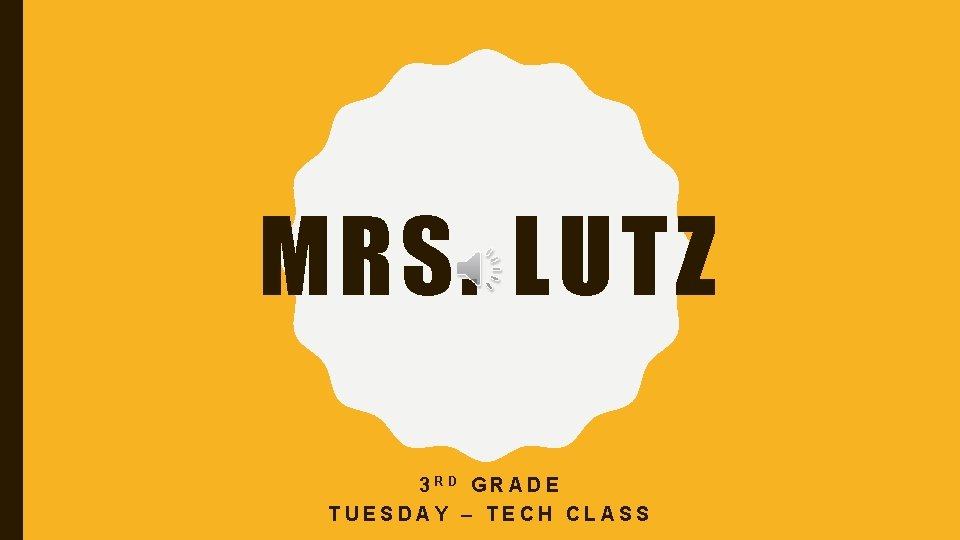MRS. LUTZ 3 RD GRADE TUESDAY – TECH CLASSSTATES OF MATTERS N I AN IM CA S T A T E S K D A O F M A T T E R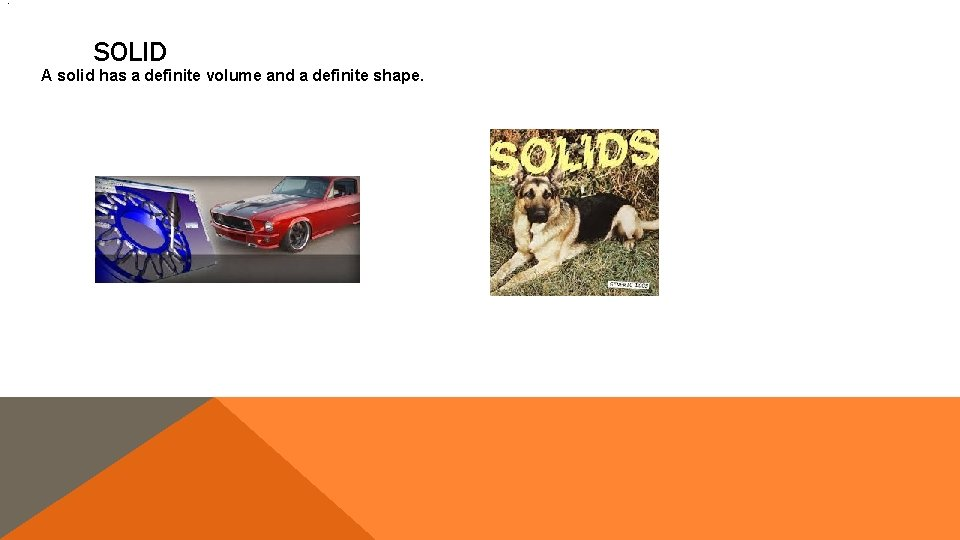. SOLID A solid has a definite volume and a definite shape.LIQUID A liquid has a definite volume but no definite shape. Liquids take the shape of the container they are in.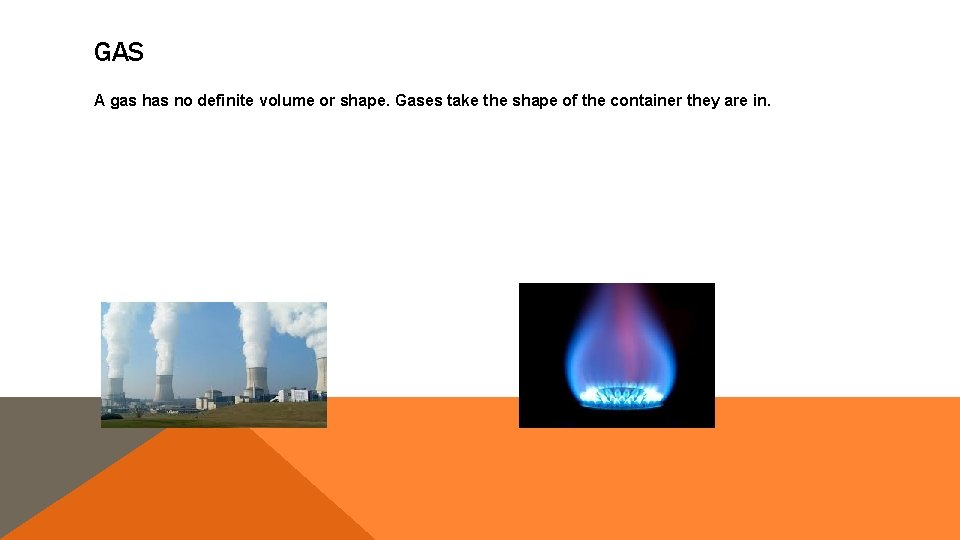GAS A gas has no definite volume or shape. Gases take the shape of the container they are in.THE ENDHARLEY BURTON States of matter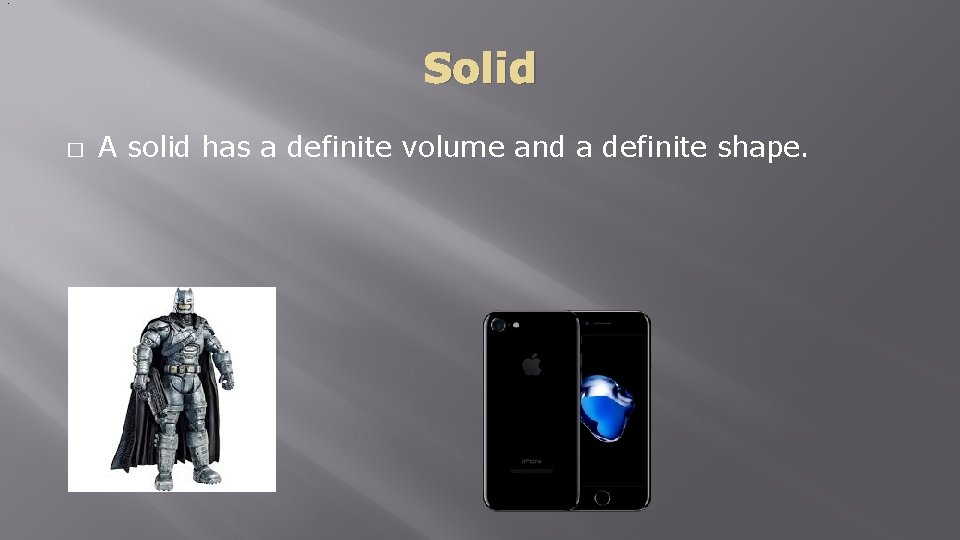. Solid � A solid has a definite volume and a definite shape.Liquid � � A liquid has a definite volume but no definite shape. Liquids take the shape of the container they are in.Gas � A gas has no definite volume or shape. Gases take the shape of the container they are in.THE ENDJA D E R S T A S ER A T P T A O M C F T E S O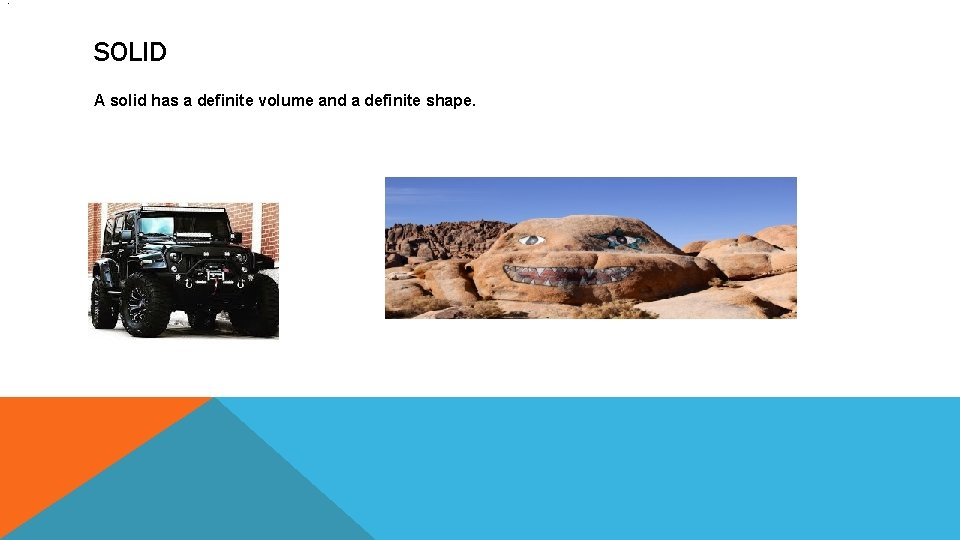. SOLID A solid has a definite volume and a definite shape.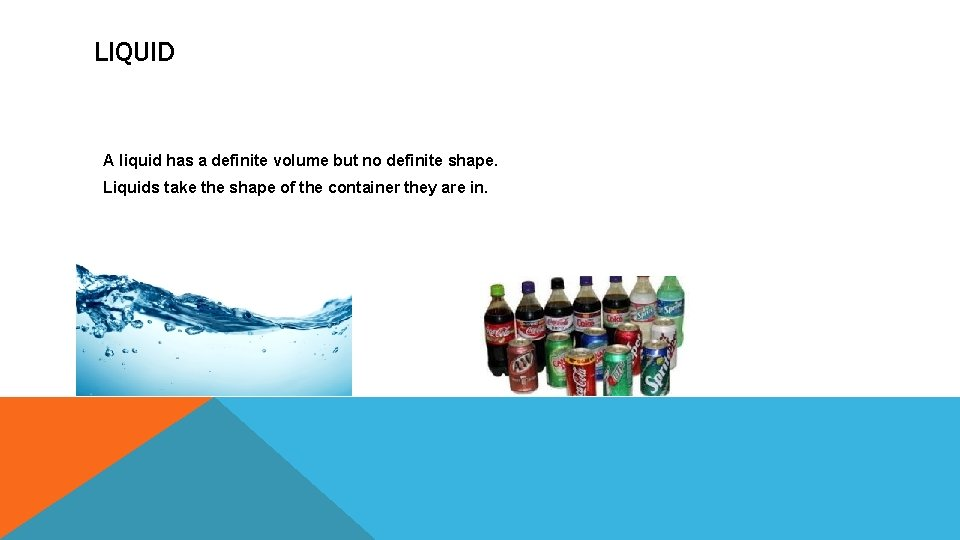LIQUID A liquid has a definite volume but no definite shape. Liquids take the shape of the container they are in.GAS A gas has no definite volume or shape. Gases take the shape of the container they are in.THE ENDBrady Doss States of matter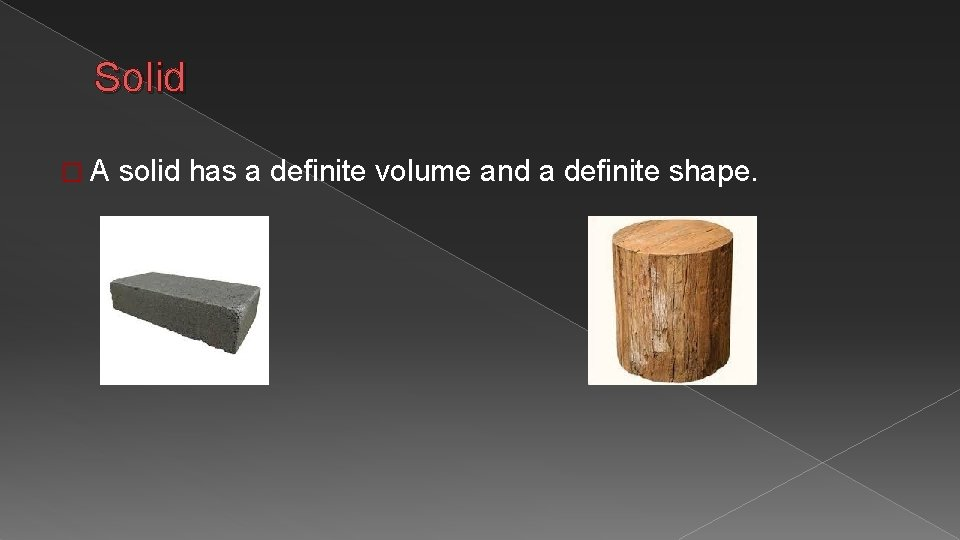. Solid �A solid has a definite volume and a definite shape.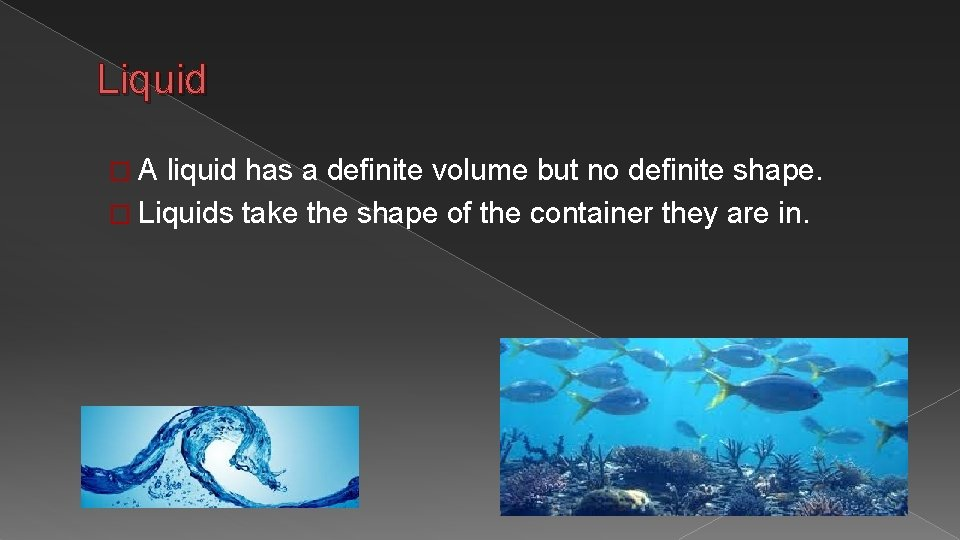Liquid �A liquid has a definite volume but no definite shape. � Liquids take the shape of the container they are in.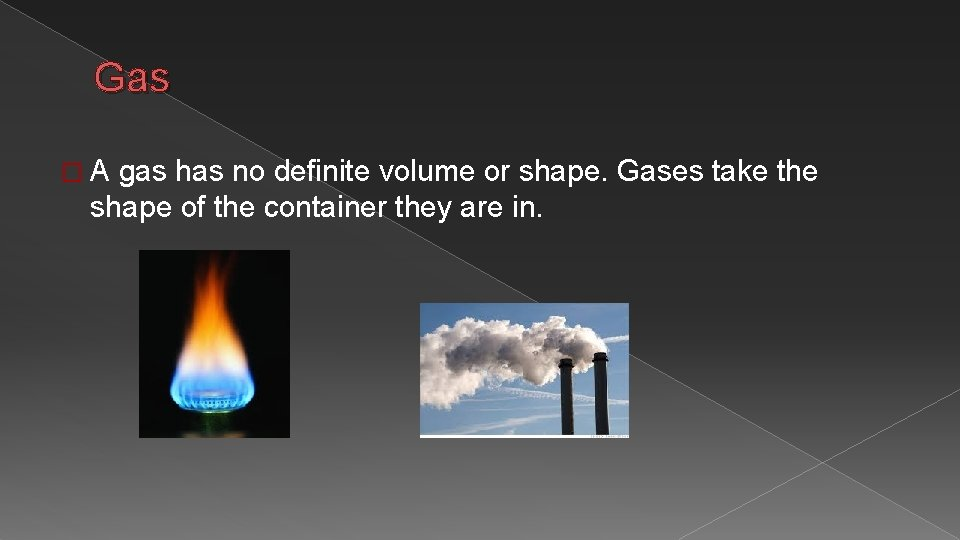Gas �A gas has no definite volume or shape. Gases take the shape of the container they are in.The end END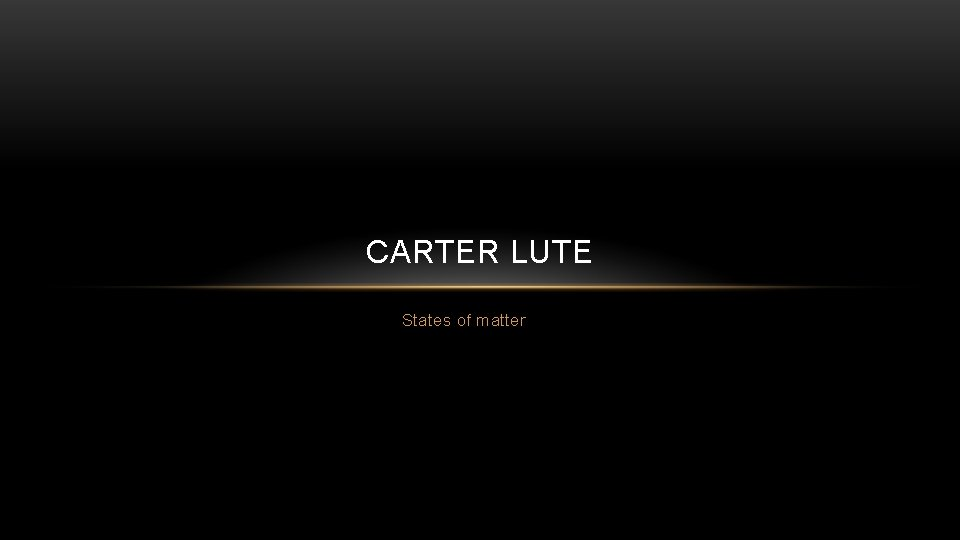CARTER LUTE States of matter. SOLID • A solid has a definite volume and a definite shape.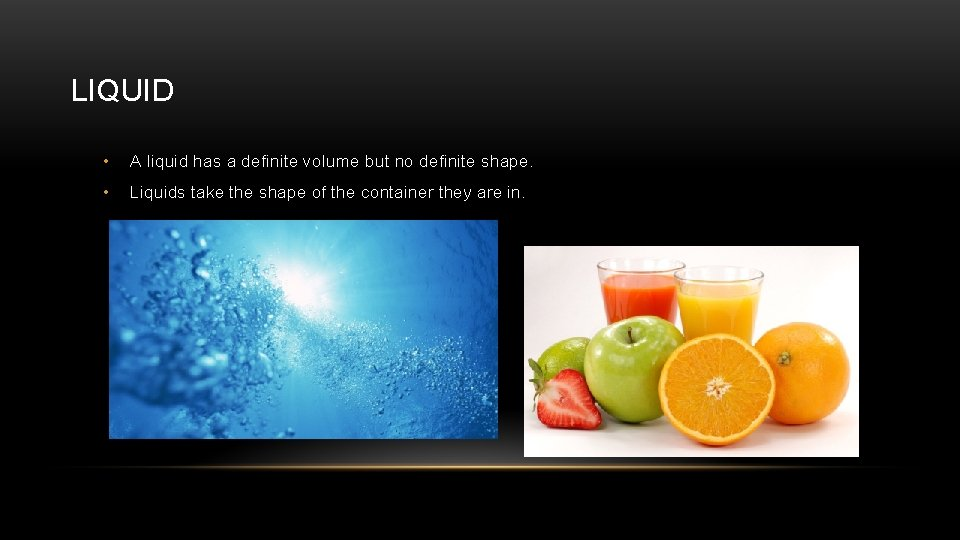LIQUID • A liquid has a definite volume but no definite shape. • Liquids take the shape of the container they are in.GAS • A gas has no definite volume or shape. Gases take the shape of the container they are in.THE ENDLogan Wolfenbarker States of matter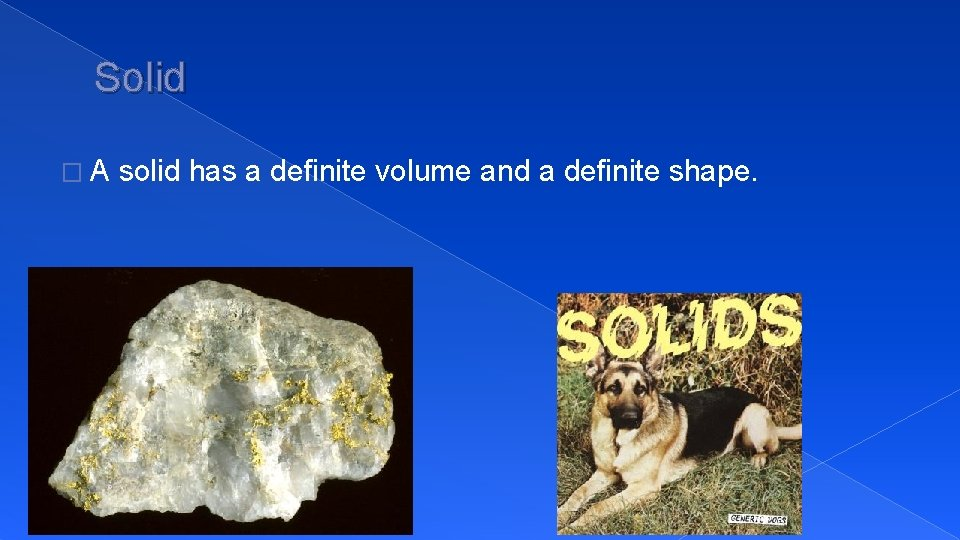. Solid �A solid has a definite volume and a definite shape.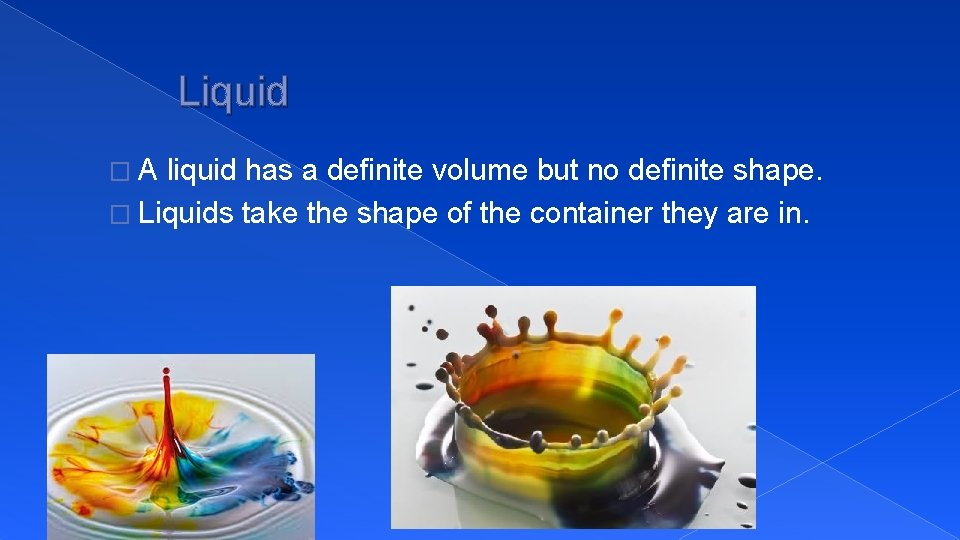Liquid �A liquid has a definite volume but no definite shape. � Liquids take the shape of the container they are in.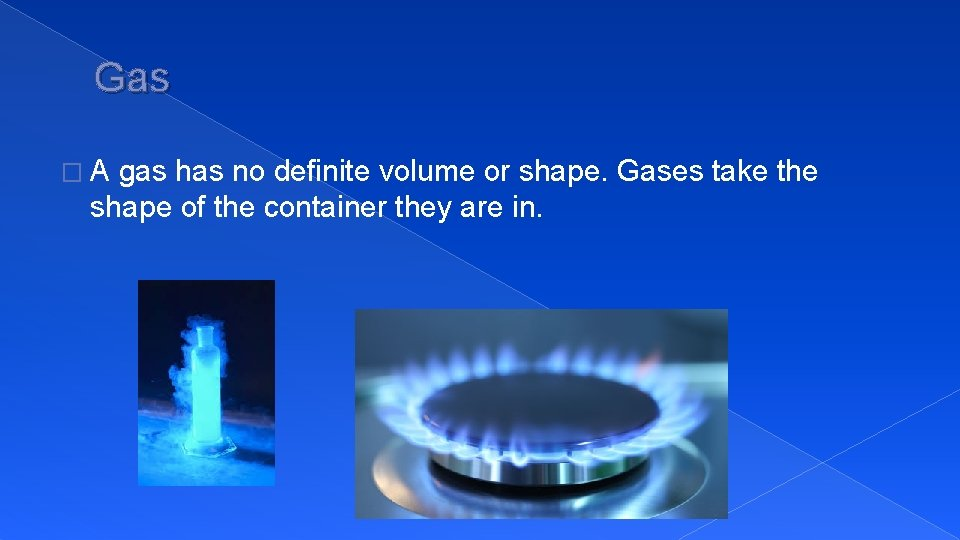Gas �A gas has no definite volume or shape. Gases take the shape of the container they are in.THE ENDStates of matter JENNA BOWLING. Solid A solid has a definite volume and a definite shape.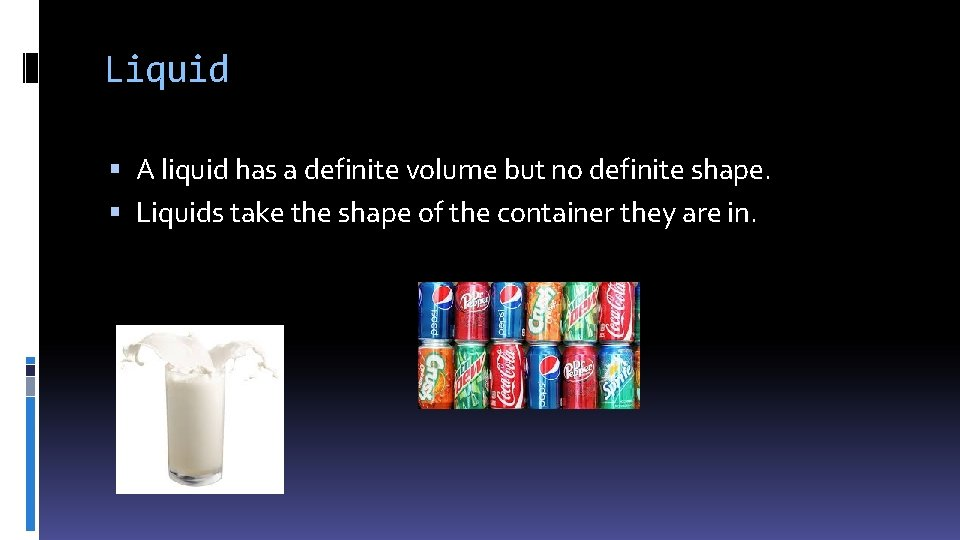Liquid A liquid has a definite volume but no definite shape. Liquids take the shape of the container they are in.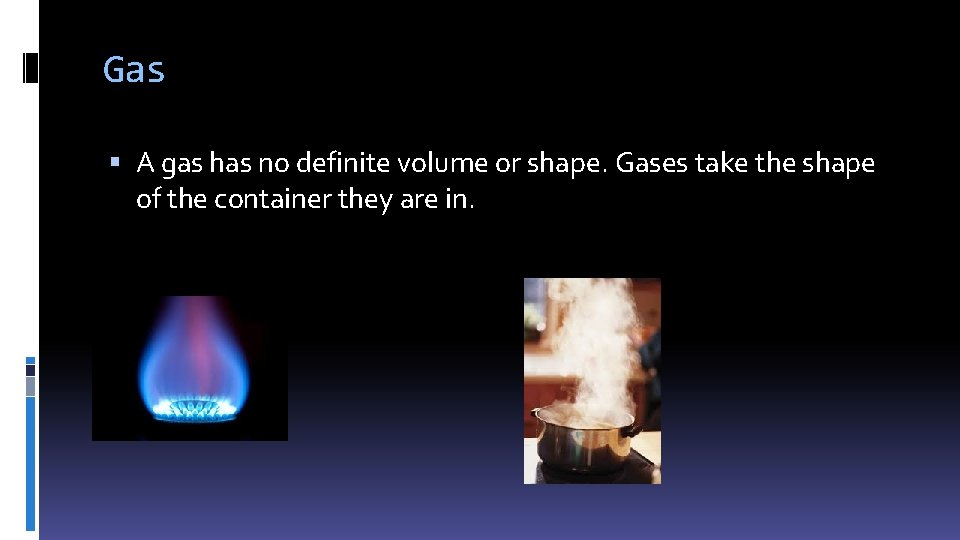Gas A gas has no definite volume or shape. Gases take the shape of the container they are in.THE ENDETHAN FERGUSON States of matter. Solid � A solid has a definite volume and a definite shape.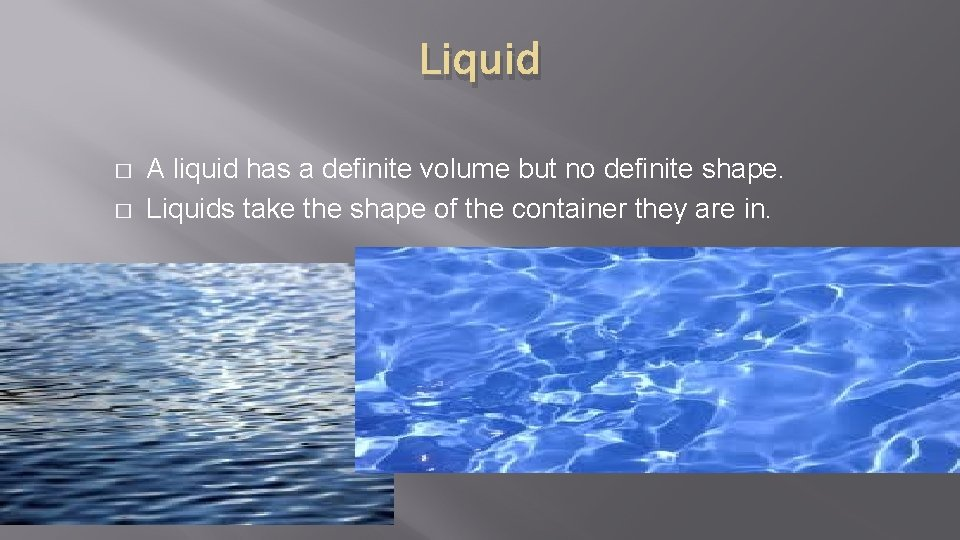Liquid � � A liquid has a definite volume but no definite shape. Liquids take the shape of the container they are in.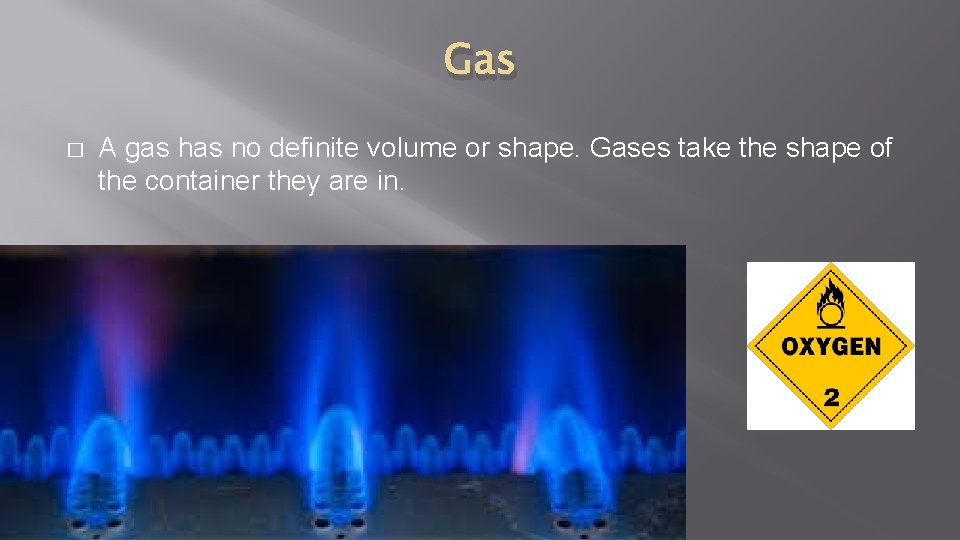Gas � A gas has no definite volume or shape. Gases take the shape of the container they are in.THE END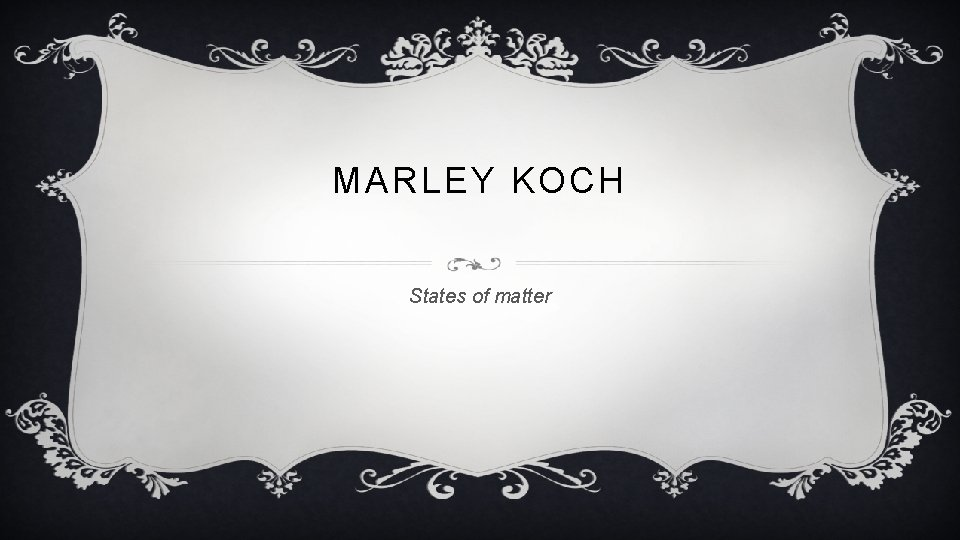MARLEY KOCH States of matter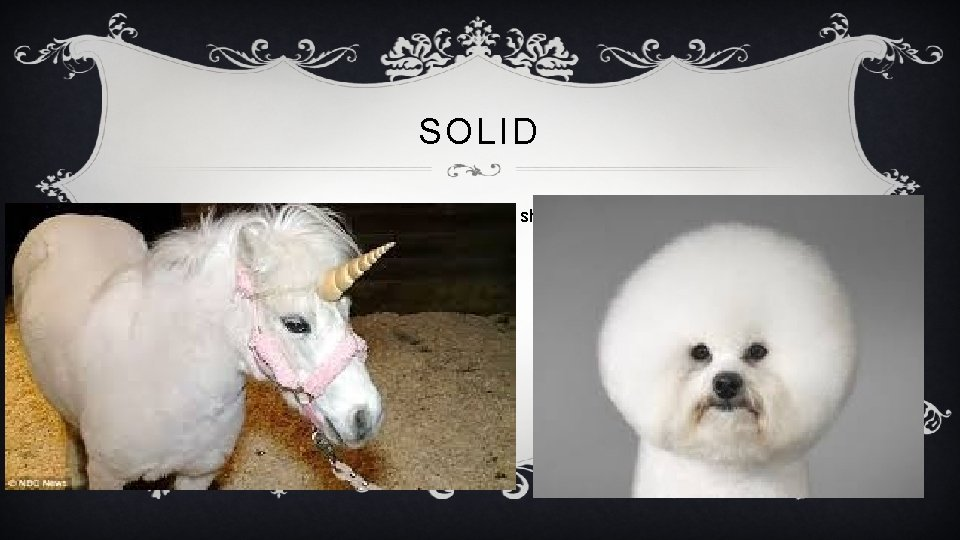. SOLID v A solid has a definite volume and a definite shape.LIQUID v A liquid has a definite volume but no definite shape. v Liquids take the shape of the container they are in.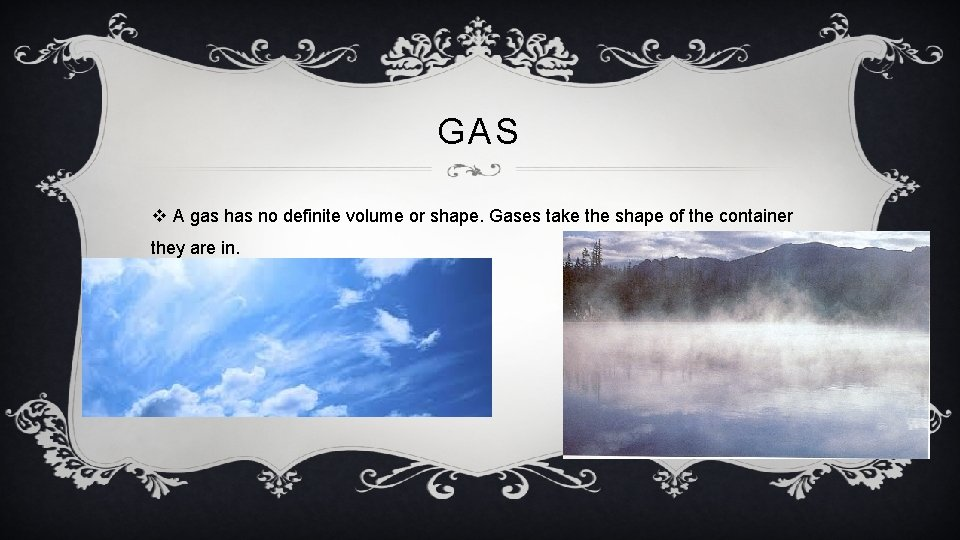GAS v A gas has no definite volume or shape. Gases take the shape of the container they are in.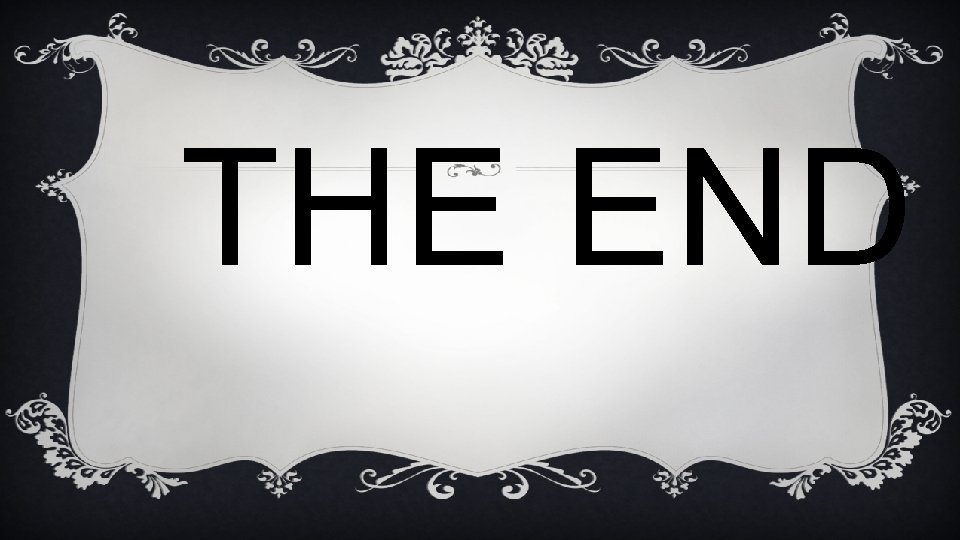THE ENDL L A H S R A M N ES A E AT S T O F M A T T E R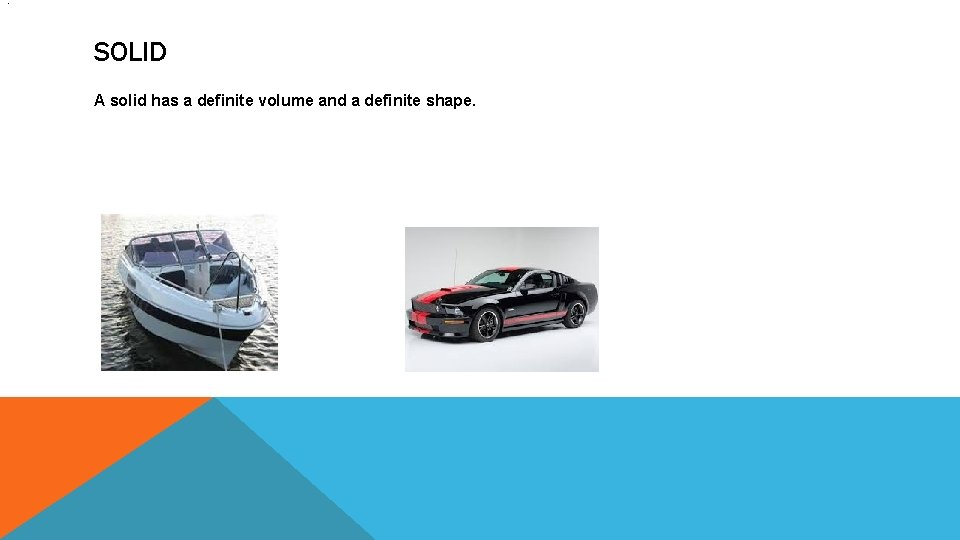. SOLID A solid has a definite volume and a definite shape.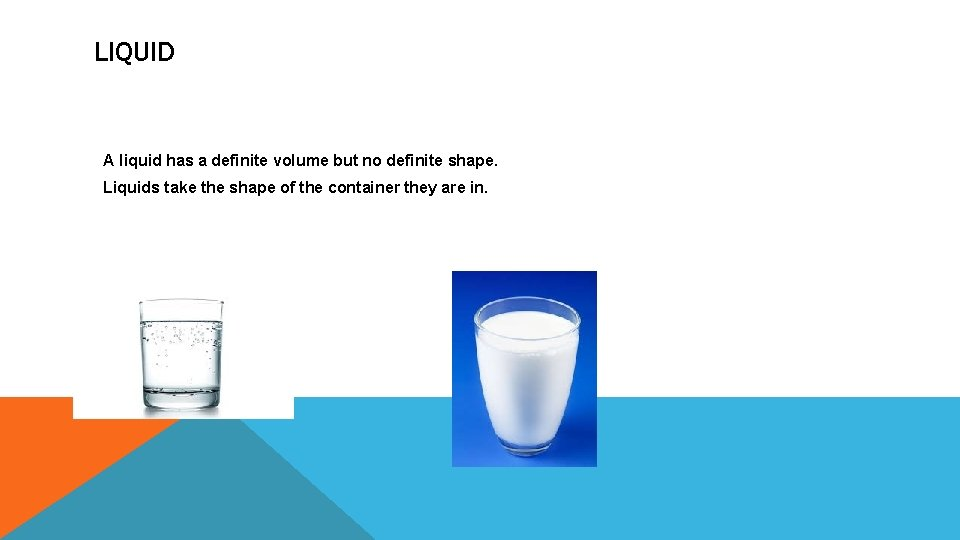LIQUID A liquid has a definite volume but no definite shape. Liquids take the shape of the container they are in.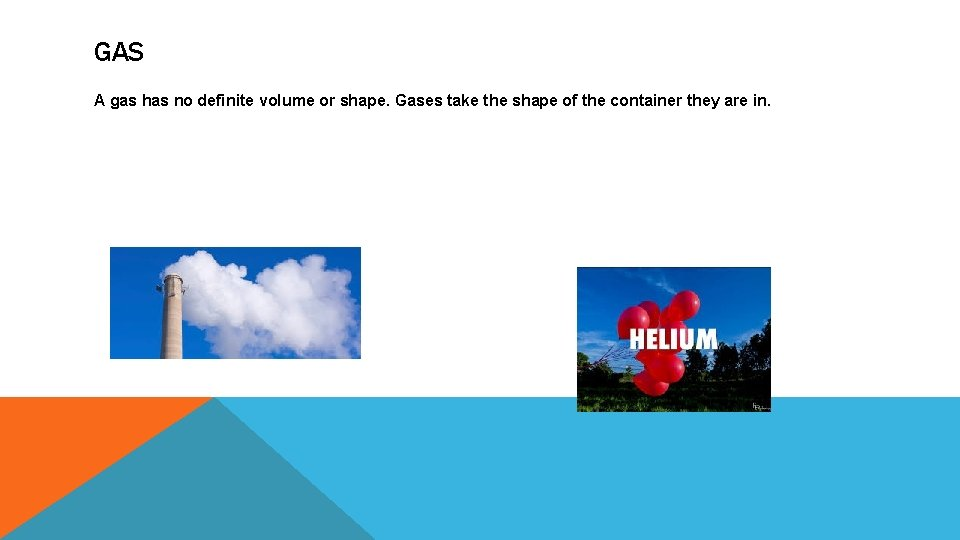GAS A gas has no definite volume or shape. Gases take the shape of the container they are in.THE ENDLILY MCINTIRE States of matter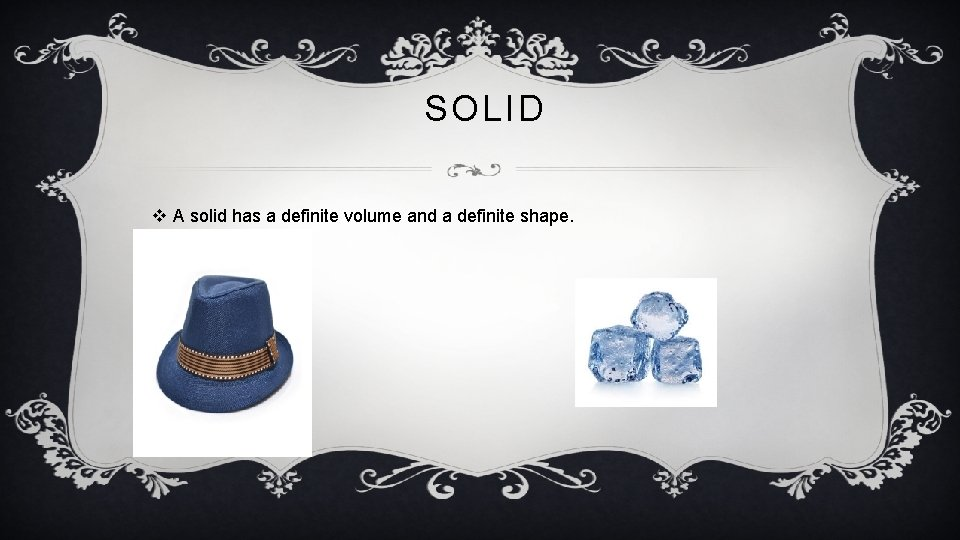. SOLID v A solid has a definite volume and a definite shape.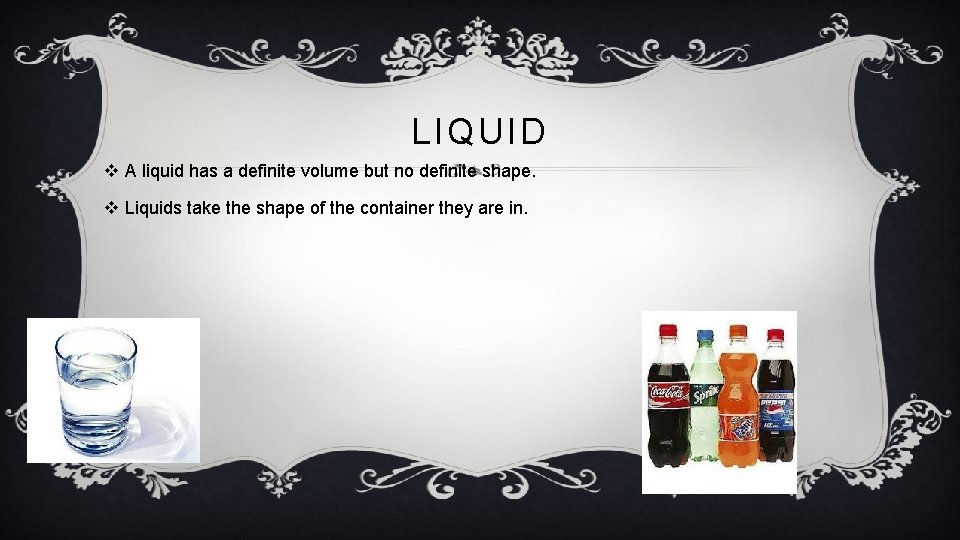LIQUID v A liquid has a definite volume but no definite shape. v Liquids take the shape of the container they are in.GAS v A gas has no definite volume or shape. Gases take the shape of the container they are in.THE ENDW B B E R T T E A H M C AT TH S T A T E S O F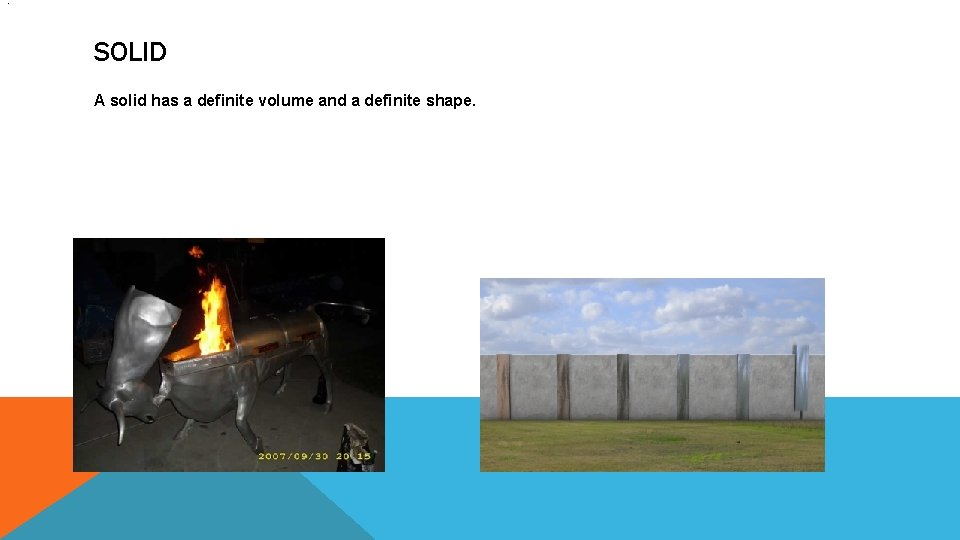. SOLID A solid has a definite volume and a definite shape.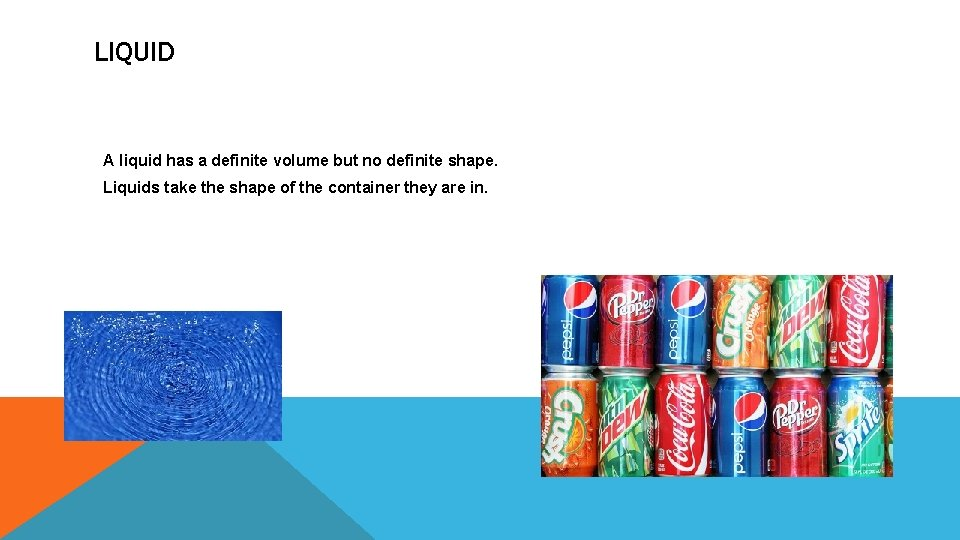LIQUID A liquid has a definite volume but no definite shape. Liquids take the shape of the container they are in.GAS A gas has no definite volume or shape. Gases take the shape of the container they are in.THE ENDK C I W T S A R D N E K S T A T E S O B O F M A T T E R. SOLID A solid has a definite volume and a definite shape.LIQUID A liquid has a definite volume but no definite shape. Liquids take the shape of the container they are in.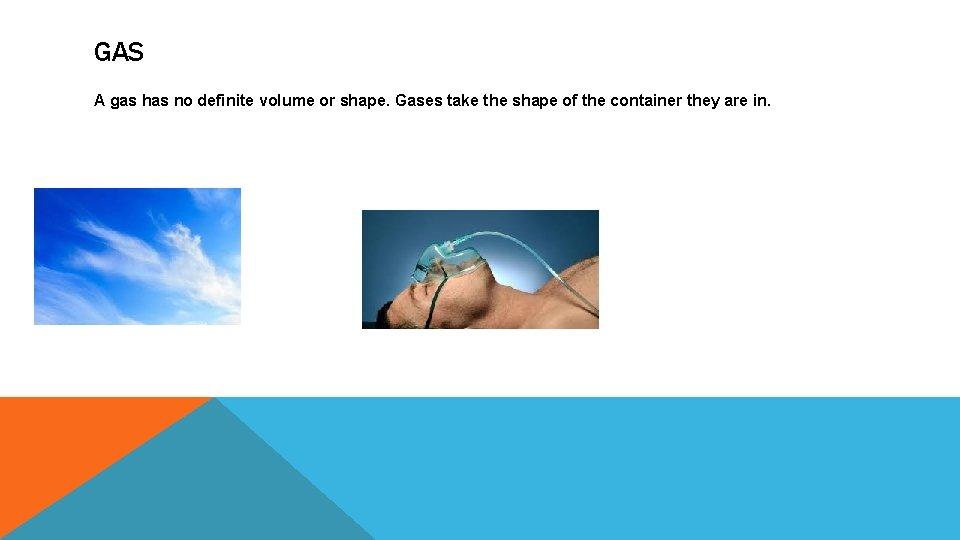GAS A gas has no definite volume or shape. Gases take the shape of the container they are in.THE ENDN E K A J S T A R E W T O T R A B T E S O F M. SOLID A solid has a definite volume and a definite shape.LIQUID A liquid has a definite volume but no definite shape. Liquids take the shape of the container they are in.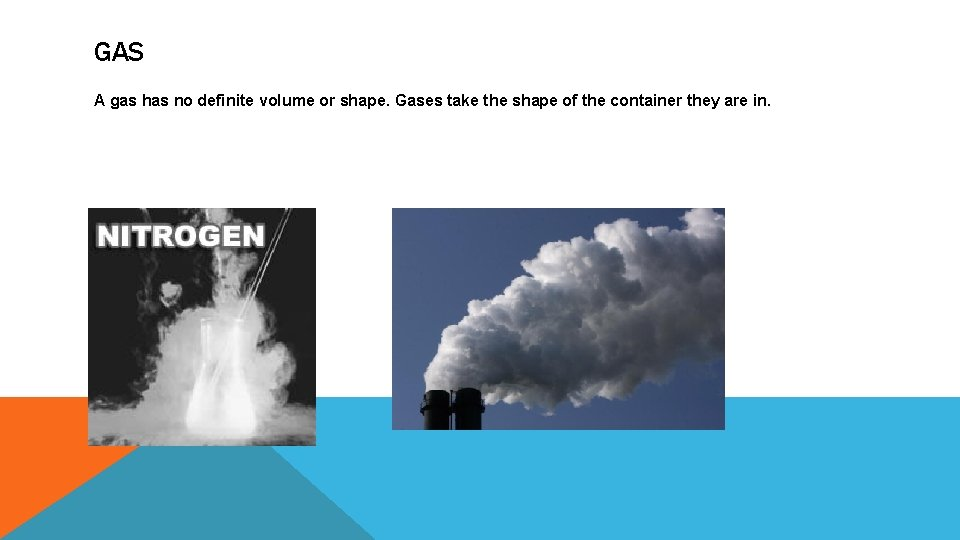GAS A gas has no definite volume or shape. Gases take the shape of the container they are in.THE END* States of matter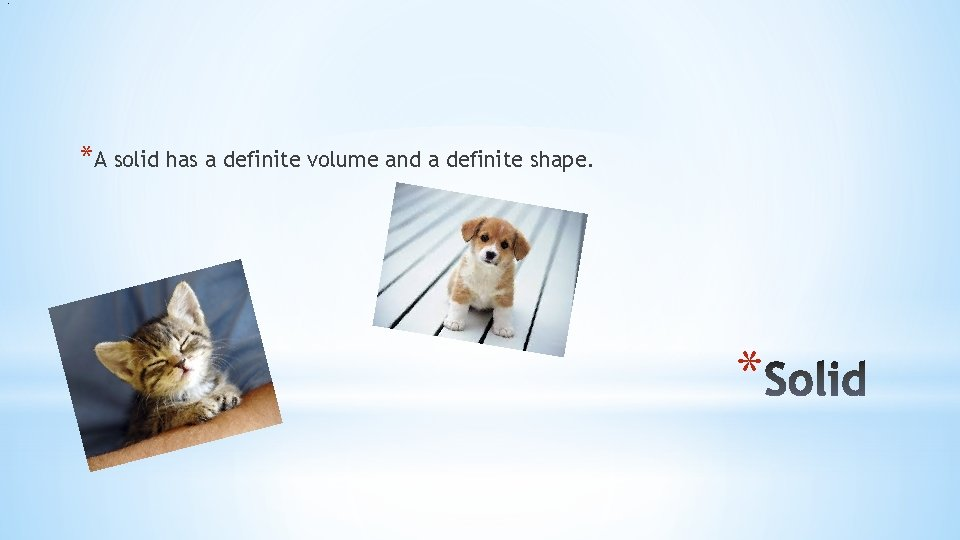. *A solid has a definite volume and a definite shape. *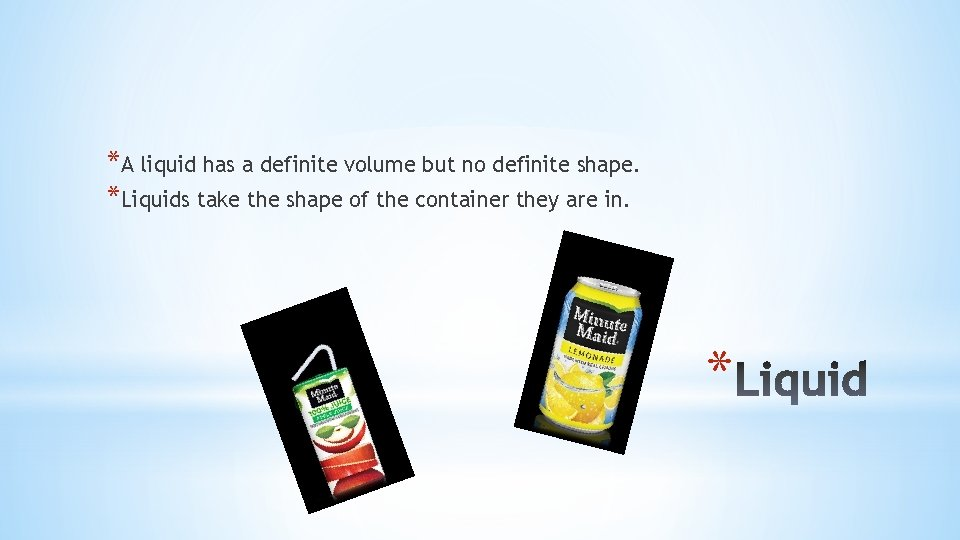*A liquid has a definite volume but no definite shape. *Liquids take the shape of the container they are in. **A gas has no definite volume or shape. Gases take the shape of the container they are in. *JAXON POWELL States of matter. Solid � A solid has a definite volume and a definite shape.Liquid � � A liquid has a definite volume but no definite shape. Liquids take the shape of the container they are in.Gas � A gas has no definite volume or shape. Gases take the shape of the container they are in.THE ENDAustin Smith States of matter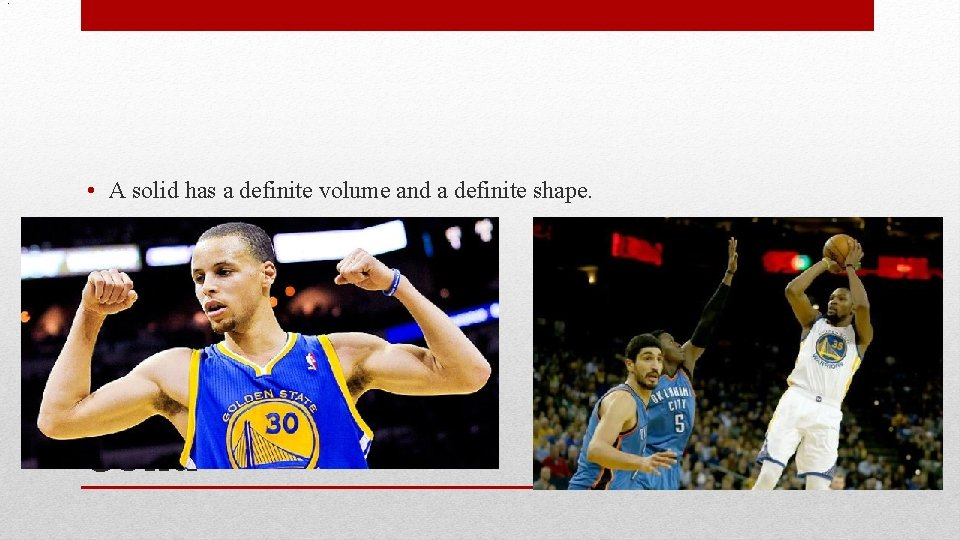. • A solid has a definite volume and a definite shape. Solid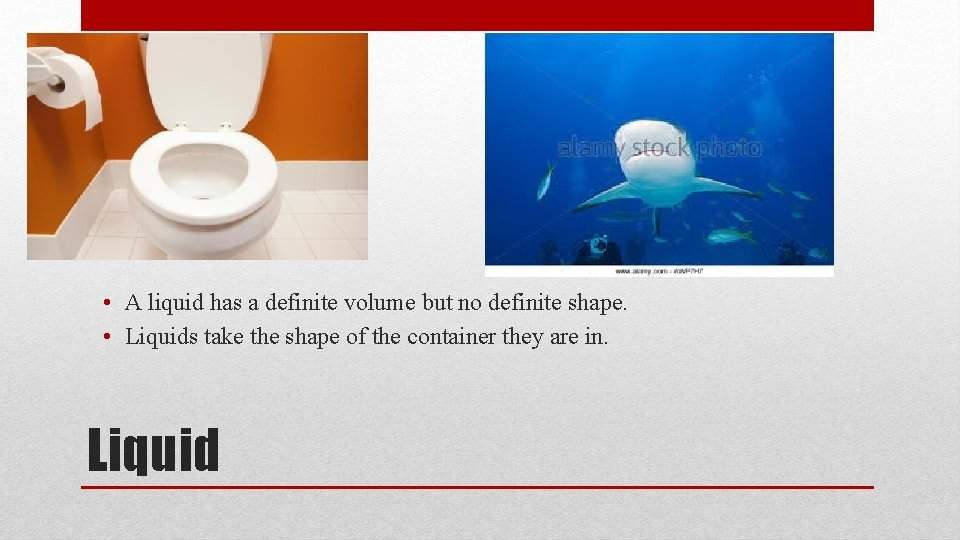• A liquid has a definite volume but no definite shape. • Liquids take the shape of the container they are in. Liquid• A gas has no definite volume or shape. Gases take the shape of the container they are in. GasTHE ENDStates of matter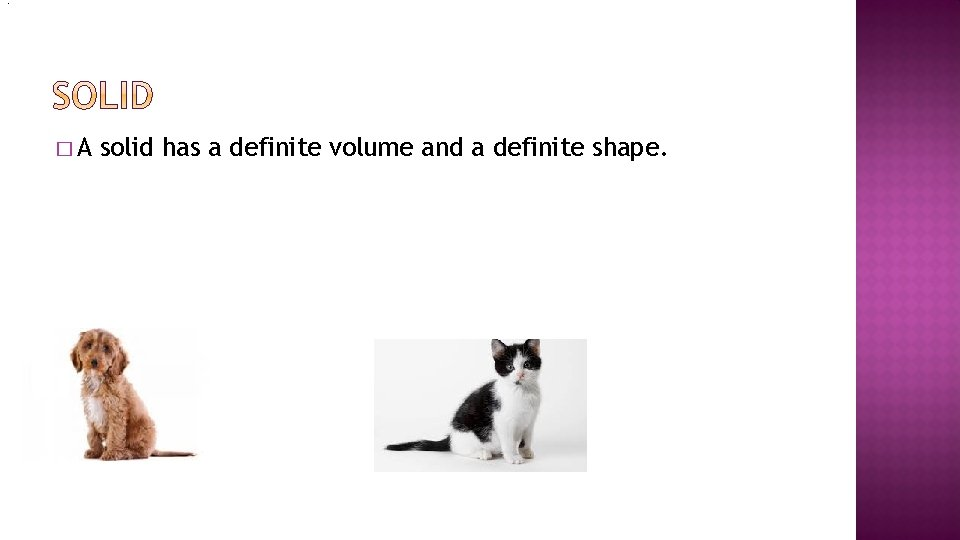. �A solid has a definite volume and a definite shape.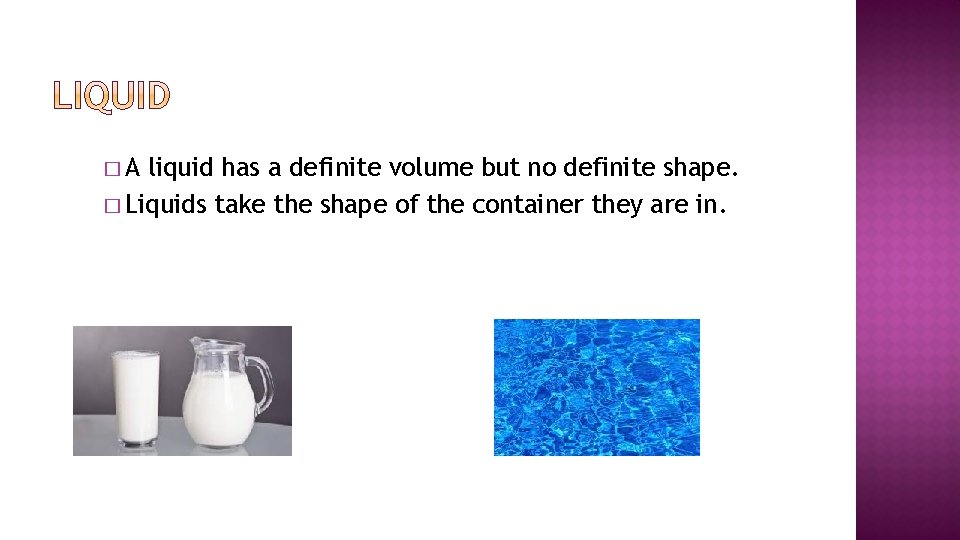�A liquid has a definite volume but no definite shape. � Liquids take the shape of the container they are in.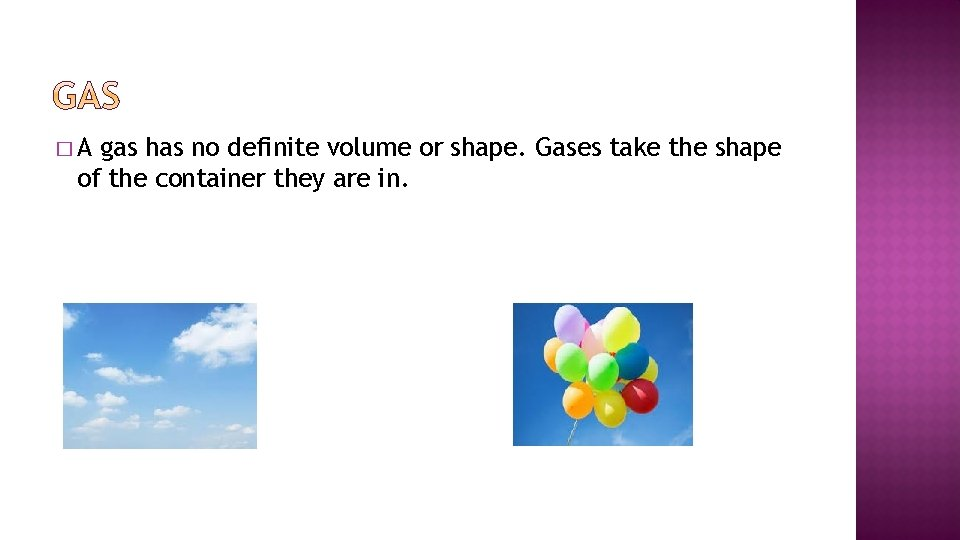�A gas has no definite volume or shape. Gases take the shape of the container they are in.